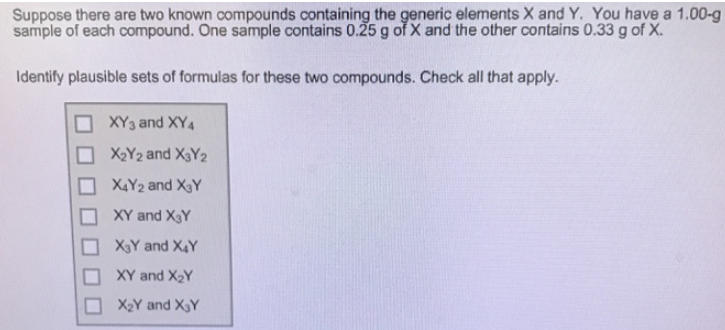# Problem: Suppose there are two known compounds containing the generic elements X and Y. You have a 1.00-g sample of each compound. One sample contains 0.25 g of X and the other contains 0.33 g of X. Identify plausible sets of formulas for these two compounds. Check all that apply.

###### FREE Expert Solution

We’re being asked to identify plausible sets of formulas for two compounds.

We’re given a 1.00-g sample of each compound. One sample contains 0.25 g of X and the other contains 0.33 g of X.

Compound 1:

mass X = 0.25 g

mass Y = 1.00 g – 0.25 g = 0.75 g

Compound 2:

mass X = 0.33 g

mass Y = 1.00 g – 0.33 g = 0.66 g

Let's take look at each option given:

XY3 and XY4

Let's use a hypothetical molar mass for X and Y to get XY3.

94% (326 ratings)###### Problem Details

Suppose there are two known compounds containing the generic elements X and Y. You have a 1.00-g sample of each compound. One sample contains 0.25 g of X and the other contains 0.33 g of X.

Identify plausible sets of formulas for these two compounds. Check all that apply.